• [奥莱理] R语言 绘图经典实例 (英文版) [奥莱理] R Graphics Cookbook (E-Book) ☆ 出版信息：☆ [作者信息] Winston Chang [出版机构] 奥莱理 [出版日期] 2013年01月03日 [图书页数] 416页 [图书语言] 英语 ...
• [Packt Publishing] R语言 绘图经典技巧 (英文版) [Packt Publishing] R Graph Cookbook (E-Book) ☆ 出版信息：☆ [作者信息] Hrishi Mittal [出版机构] Packt Publishing [出版日期] 2011年01月14日 [图书...
• 以下内容参考R语言绘图书籍：Guide to Create Beautiful Graphics in R，作者 Alboukadel Kassambara 先看个例子： data(diamonds) head(diamonds[, c("carat", "price")]) p <- ggplot(diamonds
此文内容来自微信公众号：R语言搬运工，扫码关注公众号浏览更多精彩内容、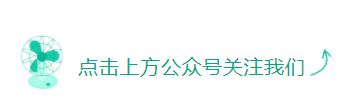绘制点密度图已成为点图增强可视化效果的重要方式，根据点的分布模式添加透明度、密度线等可以带来不一样的视觉效果。
以下内容参考R语言绘图书籍：Guide to Create Beautiful Graphics in R，作者 Alboukadel Kassambara
先看个例子：
data(diamonds)
head(diamonds[, c("carat"
展开全文• 一. R语言绘图系统在 R 里，主要有两大底层图形系统，一是 base 图形系统，二是 grid 图形系统。lattice 包与 ggplot2包正是基于 grid 图形系统构建的，它们...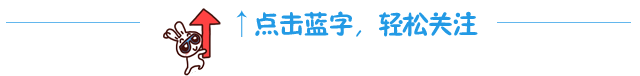一. R语言绘图系统
在 R 里，主要有两大底层图形系统，一是 base 图形系统，二是 grid 图形系统。lattice 包与 ggplot2包正是基于 grid 图形系统构建的，它们都有自己独特的图形语法。
install.packages("shiny")
install.packages("ggsci")
install.packages("esquisse")

1.base系统• 由Ross Ihaka编写• base图形是最古老的系统，在R的初期就存在• 容易上手• 修改方便，使用灵活• 不一定非常美观，需要调整参数修改• 使用较多 2.grid系统grid 图形系统可以很容易地控制图形基础单元，由Paul Murrell（2006）编写，给予编程者创作图形极大的灵活性。grid 图形系统还可以产生可编辑的图形组件，这些图形组件可以被复用和重组，并能通过 grid.layout()等函数，把图形输出到指定的位置上。但是因为 grid 包中没有提供生成统计图形及完整绘图的函数，因此很少直接采用 grid 包来分析与展示数据。3.lattice系统lattice 包通过一维、二维或三维条件绘图，即所谓的栅栏（trellis）图来对多元变量关系进行直观展示。相比于 base()函数是直接在图形设备上绘图的，lattice()函数是返回 trellis 对象。在命令执行的时候，栅栏图会被自动打印，所以看起来就像是 lattice()函数直接完成了绘图。更多关于 base、grid 和 lattice 的语法可以参考 Murrell 和 Paul 所撰写的书籍 R graphics。4.ggplot2系统ggplot2 包则基于一种全面的图形语法，提供了一种全新的图形创建方式，这套图形语法把绘图过程归纳为数据（data）、转换（transformation）、 度量（scale）、 坐标系（coordinate）、元素（element）、指引（guide）、显示（display）等一系列独立的步骤，通过将这些步骤搭配组合，来实现个性化的统计绘图。于是，得益于该图形语法，Hadley Wickham 所开发的 ggplot2 包是如此人性化，不同于 R base基础绘图和先前的 lattice 包那样参数繁多，而是摈弃了诸多烦琐细节，并以人性化的思维进行高质量作图。在 ggplot2 包中，加号（+）的引入是革命性的，这个神奇的符号完成了一系列图形语法叠加。更多 ggplot2 的使用与学习可以参考两本关于 ggplot2 的经典书籍：ggplot2 Elegant Graphicsfor Data Analysis和 R Graphics Cookbook。一般的绘图，base+ggplot2就已经够用了，所以，我们的课程就是以这2个系统进行绘图教学。ggplot2 是一个功能强大且灵活的 R 包，由 Hadley Wickham 编写，它可以生成优雅而实用的图形。ggplot2 中的 gg 表示图形语法（grammar of graphic），这是一个通过使用“语法” 来绘图的图形概念。ggplot2 主张模块间的协调与分工，整个 ggplot2 的语法框架如图 1-6-1 所示，主要包括数据绘图部分与美化细节部分。R ggplot2 图形语法的主要特点如下所示。（1）采用图层的设计方式，有利于结构化思维实现数据可视化。有明确的起始（ggplot()开始）与终止，图层之间的叠加是靠“+”实现的，越往后，其图层越在上方。通常一条 geom_xxx()函数或 stat_xxx()函数可以绘制一个图层。（2）将表征数据和图形细节分开，能快速将图形表现出来，使创造性的绘图更加容易实现。而且通过 stat_xxx()函数将常见的统计变换融入绘图中。（3）图形美观，扩展包（extension package）丰富，有专门调整颜色（color）、字体（font）和主题（theme）等辅助包。可以帮助用户快读定制个性化的图表。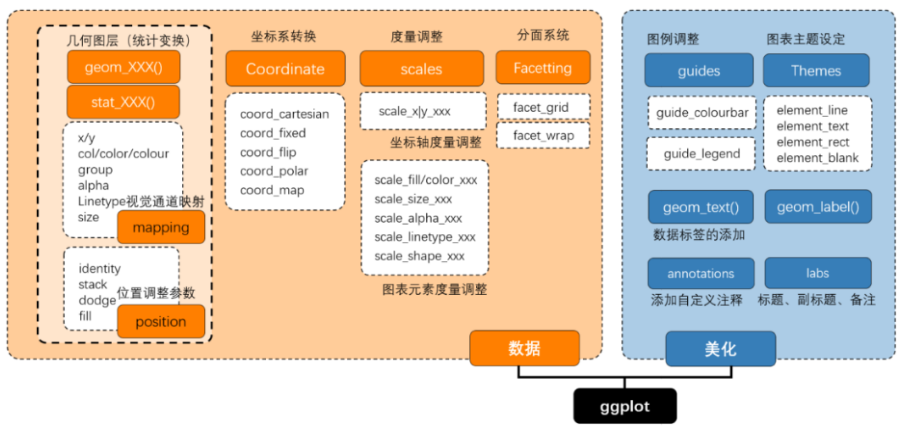ggplot2 的绘图基本语法结构如上图所示。其中所需的图表输入信息如下所示。（1）ggplot()：底层绘图函数。DATA 为数据集，主要是数据框（data.frame）格式的数据集；MAPPINGS 变量的视觉通道映射，用来表示变量 x 和 y，还可以用来控制颜色（color）、大小（size）或形状（shape）等视觉通道；STAT 表示统计变换，与 stat_xxx()相对应，默认为"identity"（无数据变换）；POSITION 表示绘图数据系列的位置调整，默认为"identity"（无位置调整）。（2）geom_xxx() | stat_xxx()：几何图层或统计变换，比如常见的 geom_point()（散点图）、geom_bar()（柱形图）、 geom_histogram()（统计直方图）、 geom_ boxplot()（箱形图）、 geom_line()（折线图）等。我们通常使用 geom_xxx()函数就可以绘制大部分图表，有时候通过设定 stat 参数可以先实现统计变换。可选的图表输入信息包括如下 5 个部分，主要是实现图表的美化与变换等。（1）scale_xxx()：度量调整，调整具体的度量，包括颜色（color）、大小（size）或形状（shape）等，跟 MAPPINGS 的映射变量相对应；（2）coord_xxx()：坐标变换，默认笛卡儿坐标系，还包括极坐标系、地理空间坐标系等；（3）facet_xxx()：分面系统，将某个变量进行分面变换，包括按行、列和网格等形式分面绘图。（4）guides()：图例调整，主要包括连续型和离散型两种类型的图例。（5）theme()：主题设定，主要用于调整图表的细节，包括图表背景颜色、网格线的间隔与颜色等。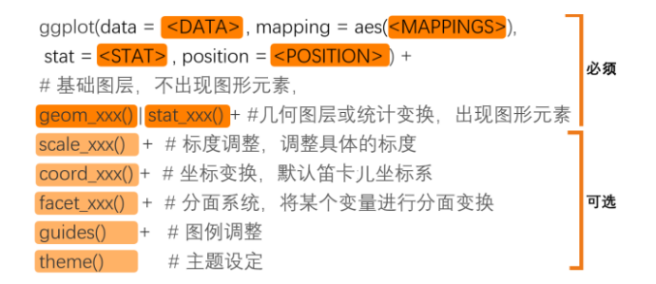二. R绘图常见函数与参数
1.低水平绘图函数
lines() 添加线
curve() 添加曲线
abline() 添加给定斜率的线
points() 添加点
segments() 折线
arrows() 箭头
axis() 坐标轴
box() 外框
title() 标题
text() 文字
mtext() 图边文字

2.高水平绘图函数
plot() 绘制散点图等多种图形
hist() 直方图
boxplot() 箱线图
stripchart() 点图
barplot() 条形图
dotplot() 点图
piechart() 饼图
interaction.plot()
matplot()

3.常用的绘图参数参数用在函数内部，在没有设定值时使用默认值。
font= 字体
lty= 线类型
lwd= 线宽度
pch= 点的类型
xlab= 横坐标
ylab= 纵坐标
xlim= 横坐标范围
ylim= 纵坐标范围
pch：指定绘制点所使用的符号，取值范围[0, 24]，其中4是“差号”，20是“点”
cex：指定符号的大小。cex是一个数值，表示pch的倍数，默认是1.5倍
lty：指定线条类型。lty=1代表实线，2至6都是虚线，虚的程度不一样
lwd：指定线条宽度，默认值为lwd=1，可以适当修改1.5倍、2倍等
col：默认绘图颜色。某些函数(如lines、pie)可以接受一个含有颜色值的向量，并自动循环使用。
例如：col=c("red", "blue")需要绘制三条线，那么三条颜色分别为red、blue、red
col.axis：坐标轴刻度文字的颜色，不是坐标轴的颜色
col.lab：坐标轴标签(名称)的颜色
col.main：标题的颜色
col.sub：副标题的颜色
fg：图形的前景色
bg：图形的背景色
cex.axis：坐标轴刻度文字的缩放倍数
cex.lab：坐标轴标签(名称)的缩放倍数
cex.main：标题的缩放倍数
cex.sub：副标题的缩放倍数
font：整数。用于指定字体样式。1常规、2粗体、3斜体、4粗斜体
pin：以英寸表示图形的宽和高
mai：以数值向量表示边界大小，顺序为"下、左、上、右"，单位为英寸
mar：以数值向量表示边界大小，顺序为"下、左、上、右"，单位为英分，默认值c(5, 4, 4, 2)+0.1
主标题可以使用函数title，格式为：title(main = " ", sub = " ", xlab = " ",  ylab = " ")

参考书籍：R语言数据可视化之美展开全文python 可视化 数据可视化 机器学习
• ## R语言基本绘图参数

万次阅读 2016-08-29 19:02:48
R语言除了具有优秀的数据处理能力外，对于数据的展现也具有极其灵活和强大的作用。 R语言绘图函数主要包括：低级绘图函数，高级绘图函数，扩展绘图包函数。 工欲善其事，必先利其器。要想绘制一副精美的...


聊一聊R的基本绘图参数

2016-03-13
砍柴问樵夫

R语言除了具有优秀的数据处理能力外，对于数据的展现也具有极其灵活和强大的作用。
R语言绘图函数主要包括：低级绘图函数，高级绘图函数，扩展绘图包函数。

工欲善其事，必先利其器。要想绘制一副精美的图形，必须掌握R中的基本绘图参数。
我们浏览一下R语言中的基本图形参数。

pch：点的符号，指定绘制点时使用的符号。
x<-rep(1:5,times=6) y<-rep(1:6,each=5) plot(0,type="n",xlim=c(1,6),ylim=c(7,1),axes=F,main='pch取值',xlab='',ylab='') for(i in 0:25){   if(i > 20){    points(x[i+1],y[i+1],pch=i,bg="red")   } else {     points(x[i+1],y[i+1],pch=i)    }   text(x[i+1]+0.4,y[i+1],labels = paste('pch=',i),cex=0.8) }
对于符号21：25，你还可以指定边界的颜色（col=）和填充颜色（bg=）。
另外，pch还可以取值：  *  . 。 +  -  |  o  等，读者自己尝试一下。

cex:指定符号的大小，一般cex 是一个数值，表示绘图符号相对于默认大小的缩放倍数。默认大小为1，1.5表示放大为默认值的1.5倍，0.5表示缩小50%。
plot(0,type="n",xlim=c(1,6),ylim=c(6,1),axes=F,      main='cex缩放倍数',xlab='',ylab='') text(x=1,y=1,labels = "cex=0.5",cex=.5) text(x=2,y=2,labels = "cex=0.8",cex=.8) text(x=3,y=3,labels = "cex=1",cex=1) text(x=4,y=4,labels = "cex=1.2",cex=1.2) text(x=5,y=5,labels = "cex=1.5",cex=1.5)
lty:指定线条类型，主要包括实线、虚线、点线、点划线等7种样式
plot(0,type="n",xlim=c(0,6),ylim=c(6,0),axes=F,      main='lty类型',xlab='',ylab='') for ( i in 0:6){   abline(h = i,lty=i)   text(x=0.1,y=i-0.1,labels = paste('lty=',i)) }

lwd：线条宽度，lwd是以默认值的相对大小来表示的（默认值为1）
plot(0,type="n",xlim=c(0,6),ylim=c(6,0),axes=F,      main='lwd宽度',xlab='',ylab='') lines(1:5,rep(1,5),lwd=0.5);text(0.5,1,labels = paste("lwd=",0.5)) lines(1:5,rep(2,5),lwd=1 );text(0.5,2,labels = paste("lwd=",1)) lines(1:5,rep(3,5),lwd=2 );text(0.5,3,labels = paste("lwd=",1.5)) lines(1:5,rep(4,5),lwd=3 );text(0.5,4,labels = paste("lwd=",2))
颜色（比较重要）
col:默认颜色向量自动循环使用
col.axis:坐标轴刻度文字的颜色
col.lab:坐标轴标签大的颜色
col.main:标题的颜色
col.sub:副标题的颜色
fg:前景色
bg:背景色
R语言默认提供了自带的固定种类的颜色。
colors()提供了657种颜色
读者可以自行选取想要的颜色
colors()[grep('red',colors())] #查找含有‘red’字符的

我们也可以将657种颜色绘制出来
pdf('1.pdf',height=100) par(mar=c(0,8,0,0)) barplot(rep(1,length(colors())),col=colors(),names.arg = colors(),horiz = T, las=1,xaxt='n') dev.off()
现将部分颜色展示如下：

R中也提供了特定颜色主题的配色方案
rainbow():彩虹的颜色
heatcolors():从红色到黄色再到白色
terrain.colors():从绿色到黄色再到棕色最后到白色
topo.colors():从蓝色到青色再到黄色最后到棕色
cm.colors():从青色到白色再到粉红色
par(mfrow=c(5,1),xaxs='i',yaxs='i',mar=c(0.1,0.1,2,0.1)) n<-1000 barplot(rep(1,n),col=rainbow(n),border=rainbow(n),axes=F,main="Rainbow()") barplot(rep(1,n),col=heat.colors(n),border=heat.colors(n),axes=F,main="heat.colors()") barplot(rep(1,n),col=terrain.colors(n),border=terrain.colors(n),axes=F,main="terrain.colors()") barplot(rep(1,n),col=topo.colors(n),border=topo.colors(n),axes=F,main="topo.colors()") barplot(rep(1,n),col=cm.colors(n),border=cm.colors(n),axes=F,main="cm.colors()")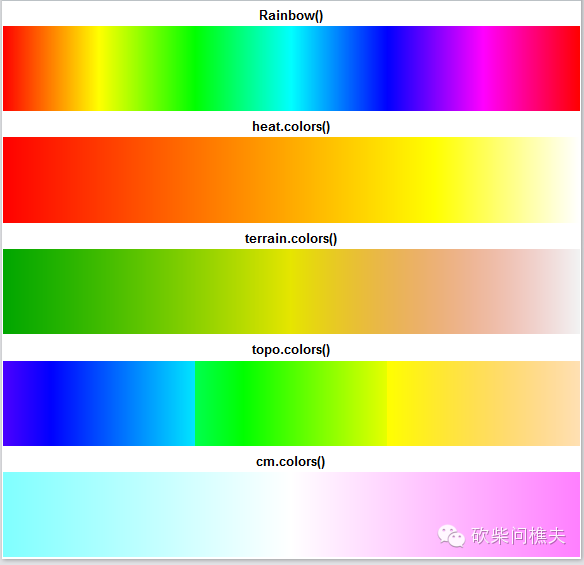此外，RcolorBrewer颜色扩展包提供了三套很好的配色方案
连续型：Sequential,通常用来标记数值的大小
极端型：生成用深色强调两端，浅色标识中部的系列颜色，用来标记数据中的离群点
离散型：生成一系列彼此差异比较明显的颜色，用来标记分类数据。
library(RColorBrewer) display.brewer.all(type="seq") #连续型 display.brewer.all(type="div") #极端性 display.brewer.all(type="qual")#离散型 display.brewer.all()           #三种类型全部输出
读者可以尝试分表输出三种类型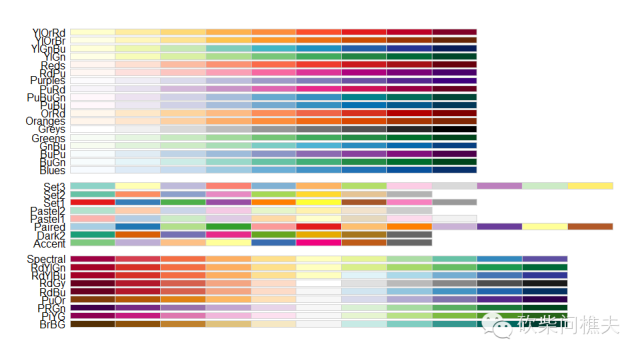文本属性：文本大小和字体

文本大小
cex:默认值为1
cex.axis:坐标轴刻度文字缩放倍数
cex.lab:坐标轴标签缩放倍数
cex.mian:标题缩放倍数
cex.sub:副标题缩放倍数
字体
font:一般用1，2，3，4，代表正常体，粗体，斜体，粗斜体
font.axis:坐标轴刻度字体样式
font.lab:坐标轴标签字体样式
font.main:标题字体样式
font.sub:副标题字体样式
ps:字体磅值
family:绘制文本时使用的字体族（serif:衬线，sans:无衬线，mono:等宽）

在window系统中，我们可以查看family的具体映射：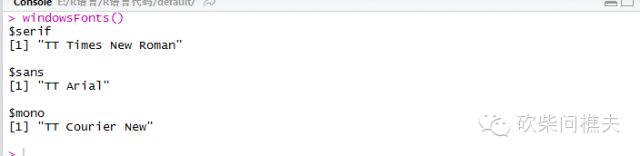如果以pdf输出图形的话，可以利用函数查看在你的系统中有哪些字体可用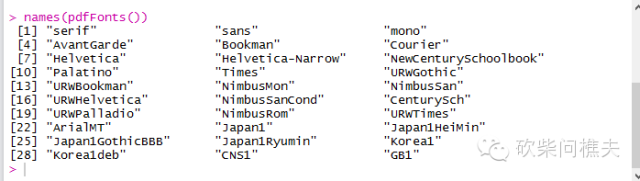查看font类型：
plot(0:5,0:5,type="n",xlab = '',ylab = '',axes = F,main="font类型") text(1,1,labels = "font=1",font=1,cex=2) text(2,2,labels = "font=2",font=2,cex=2) text(3,3,labels = "font=3",font=3,cex=2) text(4,4,labels = "font=4",font=4,cex=2)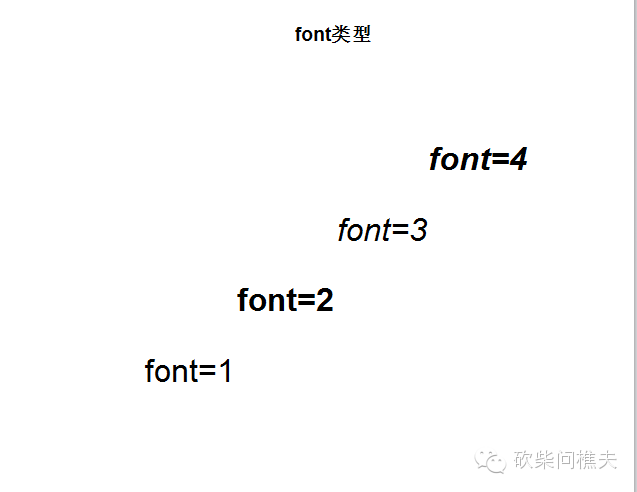图形尺寸和边界尺寸
pin:以英寸表示的图形尺寸（宽和高）
mai:以数值向量表示的边界大小（顺序是下左上右）
mar:以数值向量表示的边界大小（顺序是下左上右），默认值是c(5,4,4,2)+0.1

坐标轴
语法规则：axis(side = ,at = ,labels = ,tick = ,line = ,pos = ,outer = ,font = ,lty = ,... = )
参数 描述 side 一个整数，（1=下，2=左，3=上，4=右） at 一个数值型向量，表示需要绘制刻度线的位置 labels 一个字符型向量，表示置于刻度线旁边的文字标签（如果为NULL，则将直接使用at中的值） pos 坐标轴线绘制位置的坐标（即与另一条坐标轴相交位置的值） las 标签是否平行于（=0）或垂直于（=2）坐标轴     tck  刻度线的长度，以相对于绘图区域大小的分数表示（负值表示在图形外侧，正值表示在图形内侧，0表示禁用刻度，1表示绘制网格线）；默认值为-0.01
边框
bty参数可以设置图形边框样式，取值为 o,l,7,c,u,j
par(mfrow=c(2,3)) for(i in c('o','l','u','7','c',']')){   plot(1:10,1:10,axes=T,xlab='',ylab='',bty=i,main=paste("bty=",i)) }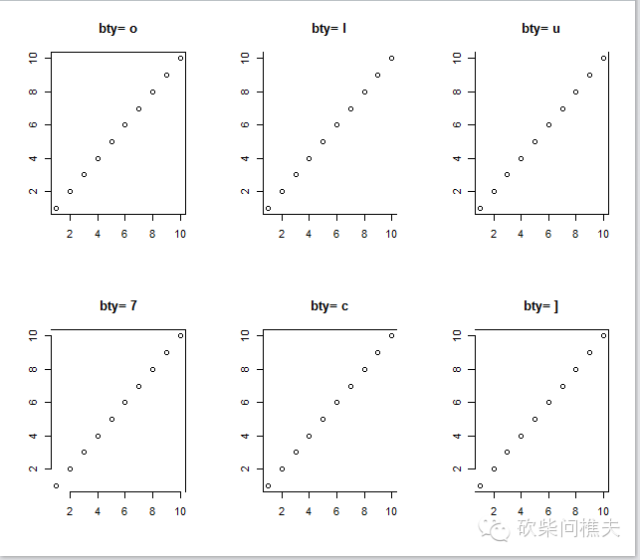当然box()函数同样可以设置各边框的线条样式

参考线
语法规则：abline(a = ,b = ,h = ,v = ,reg = ,coef = ,untf = ,... = )
a、b:截距和斜率
h、v：水平线和垂直线
coef:回归系数，提供给a、b值

网格线
语法规则：grid(nx = ,ny = ,col = ,lty = ,lwd = ,equilogs = )
nx:设置垂直网格线数目
ny：设置水平网格线数目

线段
segments()函数
语法规则：segments(x0 = ,y0 = ,x1 = ,y1 = ,col = ,lty = ,lwd = ,... = )
x0和y0表示线段的起始点，
x1和y1表示线段的终点坐标

箭头
语法规则：arrows(x0 = ,y0 = ,x1 = ,y1 = ,length = ,angle = ,code = ,col = ,lty = ,lwd = ,... = )
x0、y0、x1、y1代表绘制的起始和终点坐标
length:箭头边缘的长度
angle：箭头角度
code:在起点还是终点或者两者都绘制箭头（0：不绘制，1：起点，2：终点，3：两端）
plot(1:10,1:10,axes=F,xlab='',ylab='',type="n") arrows(1,1,8,1,angle=90,length = 0.1,code = 0);text(9,1,'angle=90') arrows(1,3,8,3,angle=60,length = 0.1,code = 1);text(9,3,'angle=60') arrows(1,5,8,5,angle=45,length = 0.1,code = 2);text(9,5,'angle=45') arrows(1,7,8,7,angle=30,length = 0.1,code =3);text(9,7,'angle=30') arrows(1,9,8,9,angle=0, length = 0.1,code = 1);text(9,9,'angle=0')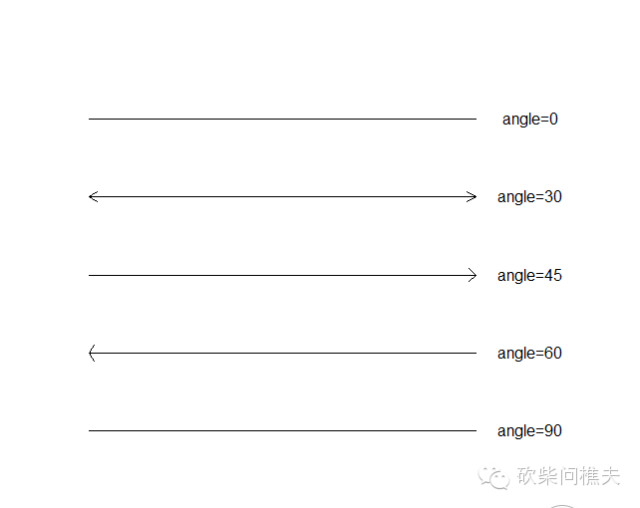type样式
在低级绘图函数中type有9中类型
par(mfrow=c(3,3)) for(i in c('p','l','b','c','o','h','s','S','n')){   plot(1:10,1:10,axes=T,xlab='',ylab='',type=i,main=paste("type=",i)) }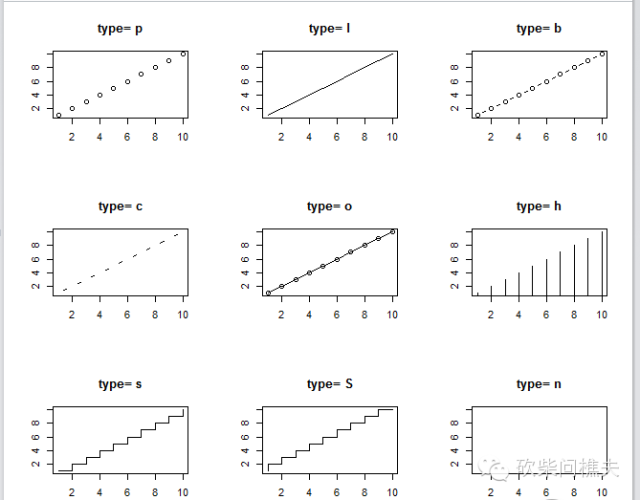图形布局
par函数和layout函数
par中图形参数mfrow=c(nrow,ncol）

layout函数更加灵活，多样。
语法规则：layout(mat = ,widths = ,heights = ,respect = )
mat：自定义的矩阵
widths:各列宽组成的向量
heights:各行高度值组成的一个向量
respect：逻辑值或者是一个矩阵对象
mat<-matrix(c(1,1,4,2,3,4),nrow = 2,byrow = T) layout(mat,widths=c(1,1,2),heights = c(1,1)) attach(mtcars) hist(mpg,main='')#高：50%，宽：50% hist(mpg,main='')#高：50%，宽：25% hist(mpg,main='')#高：50%，宽：25% hist(mpg,main='')#高：100%，宽：50%
detach(mtcars)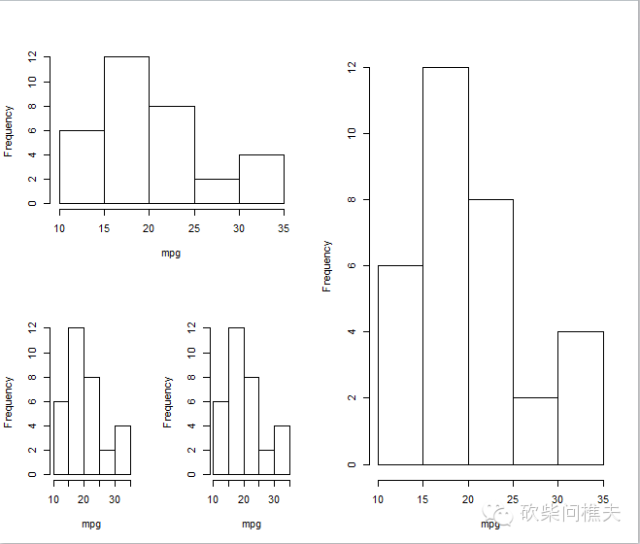若你想要精细布局你的图形，你还可以借助于fig函数
首先我们要知道一副图形，它的左下角对应的是c(0,0),右上角为c(1,1)
而fig（x1,x2,y1,y2）,x1,x2横向坐标占据空间范围，y1,y2纵向坐标范围。
opar<-par(no.readonly = T) par(fig=c(0,0.8,0.2,1)) attach(mtcars) plot(mpg~wt,xlab='') par(fig=c(0,0.8,0,0.45),new=T) boxplot(wt,horizontal = T) par(fig=c(0.7,1,0,1),new=T) boxplot(mpg) par(opar) detach(mtcars)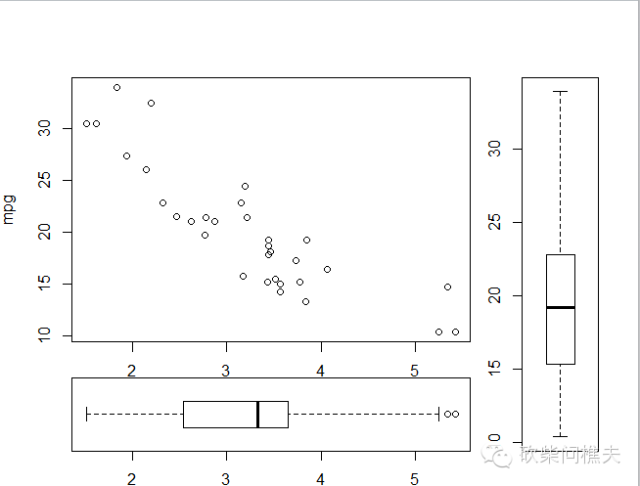R的基本绘图参数还有很多，若读者感兴趣，可以参考par函数中的内容。可以说你的par函数掌握的怎么样，就决定着你的R语言基本绘图能力如何。

参考书籍：R语言实战、R语言与网站分析


展开全文• 1 前言 为什么要使用R语言绘图？...R语言是一种编程类的统计绘图软件，主要有两大不可替代的功能：统计分析 数据作图，R语言算得上目前所有统计作图比较的一款软件.。 2 学习路线 以目前的市场上面的书籍来...
转载其他各个平台请注明作者和出处：https://blog.csdn.net/xiezhiliang22
1 前言
为什么要使用R语言绘图？数据分析界绘图界的几大巨头，Excel、Python、R，在这里就不详细讲这几者之间的关系。R语言是一种编程类的统计绘图软件，主要有两大不可替代的功能：统计分析 数据作图，R语言算得上目前所有统计作图比较的一款软件.。
2 学习路线
以目前的市场上面的书籍来看，只有为数不多的基本书籍专门讲解R语言绘图操作，都各有利弊。以个人的学习经验来看，结合以下几本书不同的章节是最快、最彻底学懂R绘图的不二之选。
NO.1 《R绘图系统》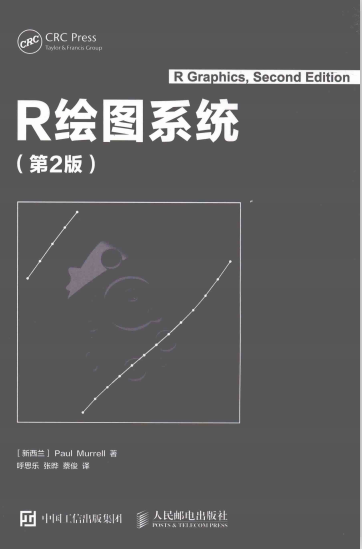利- 讲解了R各个绘图系统之间的关系，非常详细 弊-各个章节讲解了太多不适用的东西，讲解太繁琐 学习路线-只需学习书本第一章R绘图系统概况和第二章传统绘图
No.2 《R数据科学》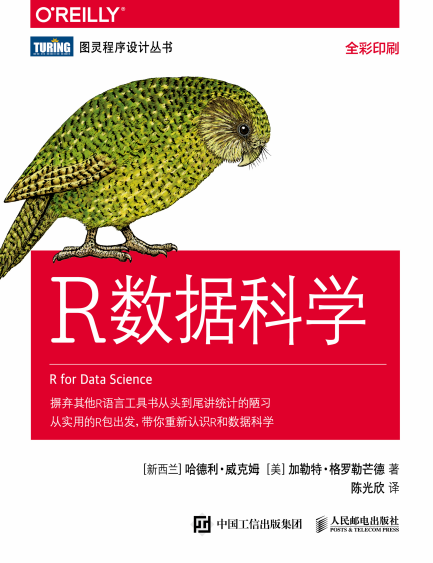利-是目前看到讲解ggplot绘图系统讲解简略易懂的最好的数 弊-本书仅仅第一章涉及到了绘图操作 学习路线-就看第一章ggplot绘图系统，搞懂习题，顺带看一下第二章基本R操作（有R语言基础可略过）
No.3 《R数据可视化手册》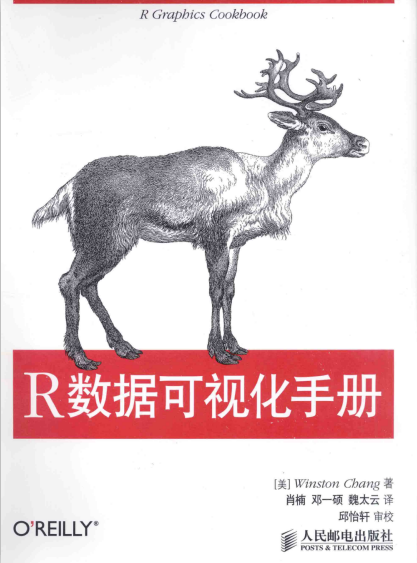利-讲解的内容算是同类书籍之中最实用的了,按照主流图形分类讲解 弊-直接上手ggplot等绘图系统，没有系统讲解ggplot等绘图系统，读者不太容易理解代码为何这样写 学习路线-是我们主要的学习书籍，绝大部分要学习
所以我们学习路线是，《R绘图系统》主要概述到《R数据科学》ggplot专题最后到《R数据可视化手册》。
此外安利一本好书应该很不错，叫《R语言数据可视化之美》，就是有点小贵，要一百多，土豪随意，不过讲解是真的不错。
3R绘图系统概述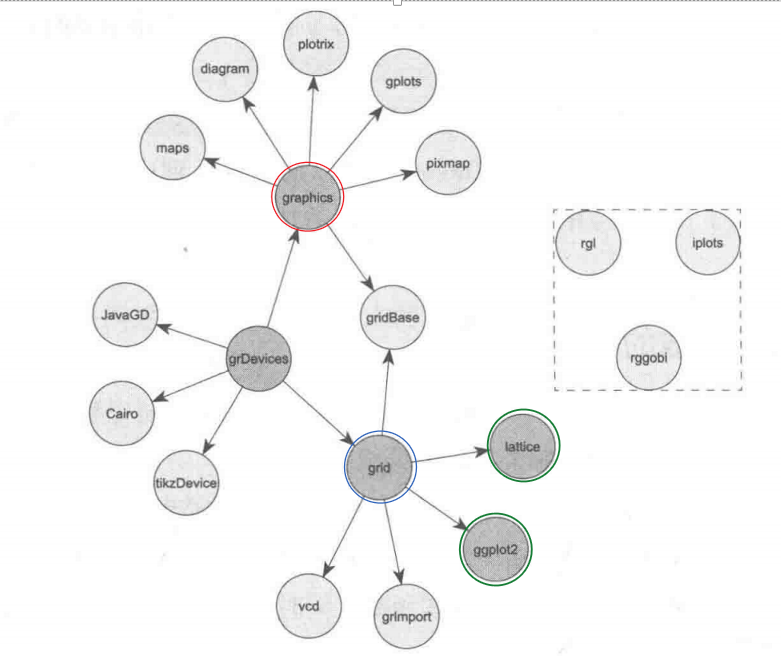红色圆圈是传统绘图，蓝色是grid系统，绿色中ggplot2是我们重点。具体的读者可以仔细阅读《R绘图系统》。
具体的书籍，CSDN里面应该有资源链接下载 接下来在专栏里会有ggplot2的概述学习总结，以及R可视化手册的总结，后续再更新。
展开全文• R语言绘图基础 WOT峰会讲师，中国R语言大会讲师，数据分析师，8年以上数据...
• 作者：黄天元，复旦大学博士在读，热爱数据科学与开源工具（R），致力于利用数据科学...书评 试读】- 京东图书，《R语言数据高效处理指南》(黄天元)【简介_书评_在线阅读】 - 当当图书）。知乎专栏：R语言数据挖掘。...
• “ 获取源代码请至公众号后台回复：一页多图”​01—图形组合布局par(mfrow=c(2,2))par(mfrow=c(2,2))，可以理解将绘图区域分割为2x2的矩阵区域，另可参照《R语言实战》3.5图形的组合##################par(mfrow=c...
• 介绍R语言的绘图基础，如固有颜色、RGB取色、主题调色板介绍，文字字体、颜色、大小等参数详解，点样式、颜色、大小等参数详解，线条样式、颜色、粗细等参数详解；详细介绍R低级绘图函数：标题、图例、坐标轴、网格...
• 参考书《R语言实战(第2版)》；作者 Robert I. Kabacoff [美]；译者 王小宁、刘撷芯、黄俊文等。建议购买正版图书学习。高密度散点图首先，感受一下什么是高密度：能看出x、y有什么关系吗？显然不能。这时候就需要...
• Constant error variance • Absence of influential cases • Linear relationship between predictors and outcome variable • Collinearity 而针对这五个假设进行验证，最直观和简单的方法就是用R语言自带的...数据分析 统计学
• ## R语言必看书籍推荐

千次阅读 2020-10-29 13:31:53
R语言实战 数据时代已经到来，但数据分析、数据挖掘人才却十分短缺。由于“大数据”对每个领域的决定性影响，　相对于经验和直觉，在商业、经济及其他领域中基于数据和分析去发现问题并作出科学、客观的决策...
• 科研绘图之R语言生存分析KM曲线和累计风险表KM估计R语言展示KM估计的生存函数曲线1、最简单的方法2、利用survminer包绘制3、进一步美化，添加累计风险表格、图例、文本注释 KM估计 KM估计同时利用生存时间（连续变量...数据挖掘 机器学习
• 本文旨在介绍R语言中ggplot2包的一些精细化操作，主要适用于对R画图有一定了解，需要更精细化作图的人，尤其是那些刚从excel转ggplot2的各位，有比较频繁的作图需求的人。不讨论那些样式非常酷炫的图表，以实用的...人工智能 python
• 本文旨在介绍R语言中ggplot2包的一些精细化操作，主要适用于对R画图有一定了解，需要更精细化作图的人，尤其是那些刚从excel转ggplot2的各位，有比较频繁的作图需求的人。不讨论那些样式非常酷炫的图表，以实用的...人工智能 python
• 数据挖掘与R语言 http://book.douban.com/subject/24153573/ 本书首先简要介绍了R软件的基础知识（安装、R数据结构、R编程、R的输入和输出等）。然后通过四个数据挖掘的实际案例 （藻类频率的预测、证券趋势预测和...R 数据挖掘 机器学习
• 那么问题来了：r语言和python有必要都学吗R:R语言由新西兰奥克兰大学的Ross Ihaka和Robert Gentleman于1995设计出来(由于两人的名字均以 ‘R’ 字母开头，因此命名为R语言)，现在由“R核心开发团队“负责开发。...
• R简介 开源，类似MATLAB，偏重于数学计算。 环境安装 工欲善其事，必先利其器。 2.1下载安装 官网地址：https://cran.r-project.org 下载地址：https://cran.r-project.org/mirrors.html，这里很感动啊，目前有...安装 基本操作
• 什么是R语言?R语言，一种自由软件编程语言与操作环境，主要用于统计分析、绘图、数据挖掘。R本来是由来自新西兰奥克兰大学的罗斯·伊哈卡和罗伯特·杰特曼开发(也因此称为R)，现在由“R开发核心团队”负责开发。R...
• R语言爬虫-高评分图书（豆瓣） # R语言爬虫-高评分图书（豆瓣） 本篇文章依然延续之前的爬虫类型文章，多次实操有助于对于代码的理解和技术的提升。此次爬取的是豆瓣上高评分的图书，每一次爬取都会给大家提供...多线程计算 RCurl爬虫 正则表达式...